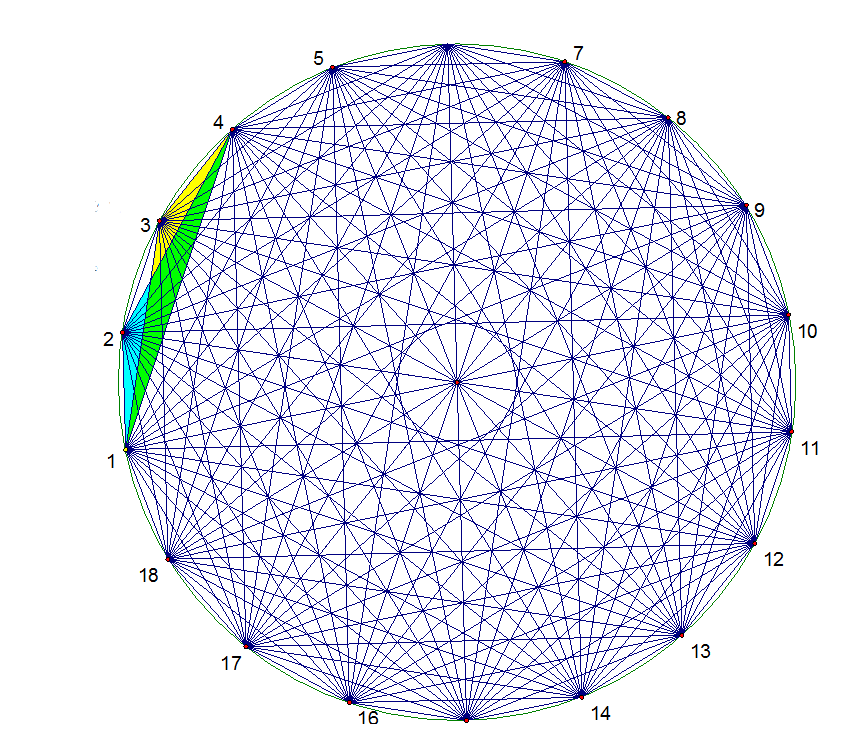# Brilliant's logo made a whole lot complex

Geometry Level 4The diagram above shows a regular $18$-gon. What is the sum of measures of the two angles, $\angle 124 + \angle 134$?